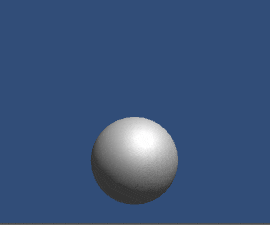### What Is A Fractal?

Fractals are non-regular geometric shapes that are self similar. Self-similar means that a smaller section of it resembles the larger whole.
These fractals can also be described as never ending patterns, as the smaller the scale we go the same patterns keep emerging.
These fractal  patterns are one of the few things that is easy to explain & to understand but very difficult to implement in such a way that the end result doesn't look like it was made by your 6 month old nephew.An Example Of A Fractal
Some famous fractal are the Mandelbrot Set & the Sierpinski Triangle.
A lot of things in nature display fractal like properties.An example of a fractal in nature

### How To Make Them In Unity?

There are several approaches that we an take to make a Fractal, Here we will generate them recursively.
So each time a new object is created it creates it's own children which then generate their own children... so on and so forth.
This is what we will end up with:Recursively creating children to make fractal
We will look at the source code and then later break down reach part.
``````using UnityEngine;
using System.Collections;

public class Fractal : MonoBehaviour
{
public Mesh mesh;
public Material material;
public int maxDepth;
public float childScale;

private int currentDepth;

private void Start()
{
if (currentDepth < maxDepth)
}

{
yield return new WaitForSeconds(0.5f);
Initialize(this, Vector3.up, Quaternion.identity);
Initialize(this, Vector3.right, Quaternion.Euler(0f, 0f, -90f));
Initialize(this, Vector3.left, Quaternion.Euler(0f, 0f, 90f));
}

private void Initialize(Fractal parent, Vector3 direction, Quaternion orientation)
{
mesh = parent.mesh;
material = parent.material;
maxDepth = parent.maxDepth;
currentDepth = parent.currentDepth + 1;
childScale = parent.childScale;
transform.parent = parent.transform;
transform.localScale = Vector3.one * childScale;
transform.localPosition = direction * (0.5f + 0.5f * childScale);
transform.localRotation = orientation;
}
} ``````
Let's go through the first part:
``````public Mesh mesh;
public Material material;
public int maxDepth;
public float childScale;

private int currentDepth;

private void Start()
{
if (currentDepth < maxDepth)
}``````
Each time an object is instantiated we call the start function and there we will provide what ever mesh and material is supposed to be assigned to the object. In our case we used the default unity Sphere mesh.
Also we have to prevent anymore object instantiation after a certain depth otherwise things can get out of hand.😜.
Now we will look at the 2nd Part:
``````private IEnumerator AddChild()
{
yield return new WaitForSeconds(0.5f);
Initialize(this, Vector3.up, Quaternion.identity);
Initialize(this, Vector3.right, Quaternion.Euler(0f, 0f, -90f));
Initialize(this, Vector3.left, Quaternion.Euler(0f, 0f, 90f));
}
``````
After the object has been instantiated, it's start function will call this Co-routine and create 3 new game objects one at each side of the parent, except for the bottom side.
Now for the juicy part:
``````private void Initialize(Fractal parent, Vector3 direction, Quaternion orientation)
{
mesh = parent.mesh;
material = parent.material;
maxDepth = parent.maxDepth;
currentDepth = parent.currentDepth + 1;
childScale = parent.childScale;
transform.parent = parent.transform;
transform.localScale = Vector3.one * childScale;
transform.localPosition = direction * (0.5f + 0.5f * childScale);
transform.localRotation = orientation;
}``````
Every time a new fractal child is made, it's properties have to be set.
It takes the mesh and material of the parent as well as information as to what is the maximum depth of recursion.
We have to update what level of depth the current fractal child is at as well.
We then have to modify the shape of the child object, here we are just scaling it by the 'childScale' factor and we have to provide new location as well as new rotation to the child to make sure it is placed and oriented correctly in local space.
The rotation is important because if we didn't have proper rotation assigned then it's child will be having world space rotation and therefore always produce children according to world space and not local co-ordinates.
Support Bitshift Programmer by leaving a like on Bitshift Programmer Facebook Page and be updated as soon as there is a new blog post.